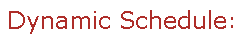Visit Minerva Class Schedule for course dates & times.

# MATH 247 Honours Applied Linear Algebra (3 credits)

Offered by: Mathematics and Statistics (Faculty of Science)

### Overview

Mathematics & Statistics (Sci) : Matrix algebra, determinants, systems of linear equations. Abstract vector spaces, inner product spaces, Fourier series. Linear transformations and their matrix representations. Eigenvalues and eigenvectors, diagonalizable and defective matrices, positive definite and semidefinite matrices. Quadratic and Hermitian forms, generalized eigenvalue problems, simultaneous reduction of quadratic forms. Applications.

Terms: Winter 2023

Instructors: Jakobson, Dmitry (Winter)

• Winter

• Prerequisite: MATH 133 or equivalent.

• Restriction: Intended for Honours Physics and Engineering students

• Restriction: Not open to students who have taken or are taking MATH 236, MATH 223 or MATH 251